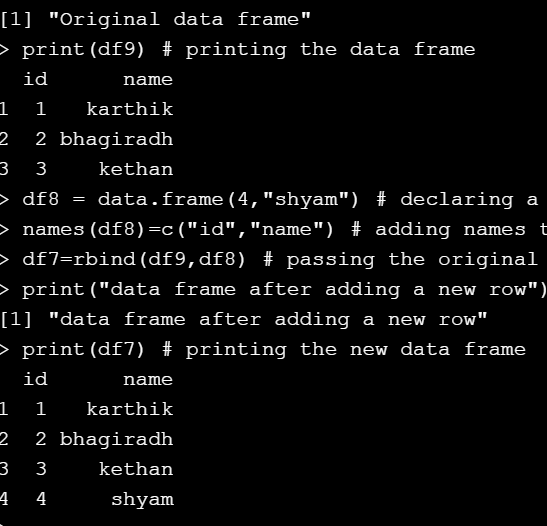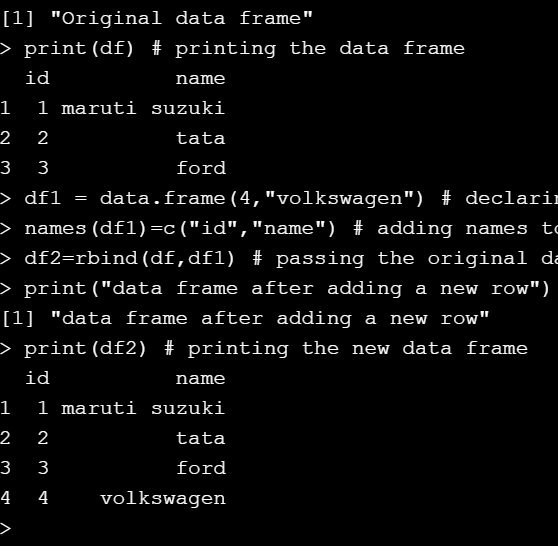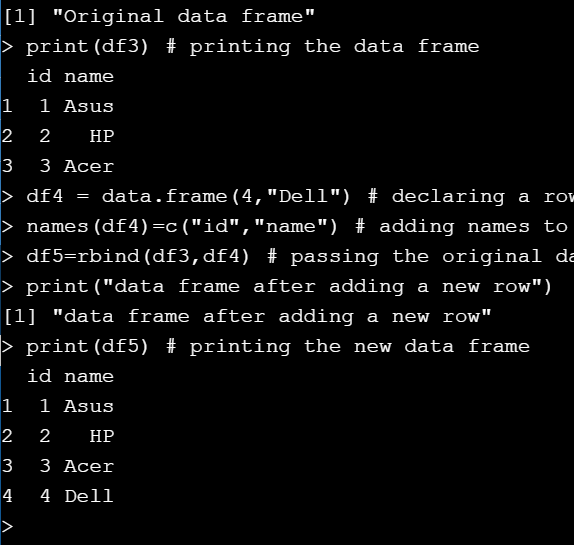# How to add a row to R dataframe ?

In this article, we will see how to add rows to a DataFrame in R Programming Language. To do this we will use rbind() function. This function in R Language is used to combine specified Vector, Matrix or Data Frame by rows.

Syntax:

rbind(dataframe 1, dataframe 2)

Example 1 :

## R

 `# creating a data frame with some data` `df9 = ``data.frame``(id=``c``(1,2,3),` `                 ``name=``c``(``"karthik"``,``"bhagiradh"``,``"kethan"``)) `   `print``(``"Original data frame"``)`   `# printing the data frame ` `print``(df9) `   `# declaring a row of values in ` `# data.frame() function` `df8 = ``data.frame``(4,``"shyam"``) `   `# adding names to the row values` `names``(df8)=``c``(``"id"``,``"name"``)`   `# passing the original data frame and new ` `# data frame into the rbind() function` `df7=``rbind``(df9,df8)  `   `print``(``"data frame after adding a new row"``)` `print``(df7)`

Output :Example 2 :

## Python3

 `# creating a data frame with some data` `df ``=` `data.frame(``id``=``c(``1``,``2``,``3``),` `                ``name``=``c(``"maruti suzuki"``,``"tata"``,``"ford"``)) `   `print``(``"Original data frame"``)` `print``(df) `   `# declaring a row of values in` `# data.frame() function` `df1 ``=` `data.frame(``4``,``"volkswagen"``)`   `# adding names to the row values` `names(df1)``=``c(``"id"``,``"name"``) `   `# passing the original data frame and ` `# new data frame into the rbind() function ` `df2``=``rbind(df,df1) `   `print``(``"data frame after adding a new row"``)` `print``(df2)`

Output :Example 3:

## R

 `# creating a data frame with some data` `df3 = ``data.frame``(id=``c``(1,2,3),` `                 ``name=``c``(``"Asus"``,``"HP"``,``"Acer"``)) `   `print``(``"Original data frame"``)` `print``(df3) `   `# declaring a row of values in ` `# data.frame() function` `df4 = ``data.frame``(4,``"Dell"``)`   `# adding names to the row values` `names``(df4)=``c``(``"id"``,``"name"``) `   `# passing the original data frame and ` `# new data frame into the rbind() function` `df5=``rbind``(df3,df4) `   `print``(``"data frame after adding a new row"``)` `print``(df5) `

Output :Whether you're preparing for your first job interview or aiming to upskill in this ever-evolving tech landscape, GeeksforGeeks Courses are your key to success. We provide top-quality content at affordable prices, all geared towards accelerating your growth in a time-bound manner. Join the millions we've already empowered, and we're here to do the same for you. Don't miss out - check it out now!

Previous
Next